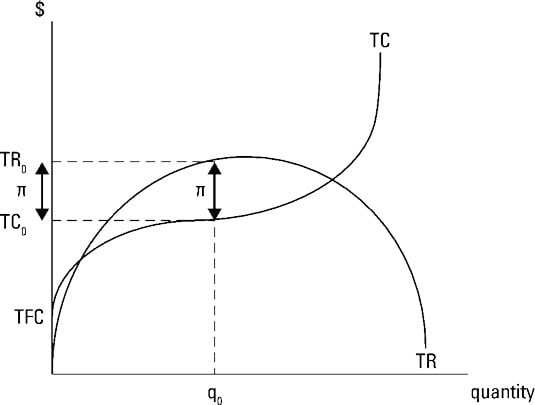##### Circular Economy For DummiesTotal profit equals total revenue minus total cost. In order to maximize total profit, you must maximize the difference between total revenue and total cost. The first thing to do is determine the profit-maximizing quantity. Substituting this quantity into the demand equation enables you to determine the good’s price. Alternatively, dividing total revenue by quantity enables you to determine price.

Graphically, the total revenue and total cost curves appear shown in the illustration. The total revenue curve increases but at a decreasing rate — the curve becomes flatter. Eventually, total revenue begins to decrease.

This relationship between total revenue and quantity reflects the fact that as a monopolist, you need to charge a lower price in order to sell more output. The lower price leads to smaller increases and eventually decreases in total revenue.The nonlinear relationship, or increasing at a decreasing rate, also reflects the changing price elasticity of demand along the demand curve. The region where total revenue is increasing corresponds to the elastic region of the market demand curve. Decreasing total revenue as output increases reflects the inelastic region of market demand where marginal revenue is negative.

Producing more in this region means less revenue because in order to sell more of the good you have to lower price on every unit you sell. The lower price on every other unit you sell decreases your revenue by more than you gain through the one extra unit you’re now able to sell. The point where total revenue is maximized corresponds to unitary elasticity.

Remember that even when you produce nothing in the short run, you’re stuck with fixed costs. So at zero units of output, the monopoly’s total fixed cost equals TFC. As the quantity of output produced increases, total cost initially increases at a decreasing rate. This situation occurs until diminishing returns begin. After diminishing returns start, total cost increases at an increasing rate. That is, the total cost curve becomes steeper.

Total profit is represented by the vertical difference between the total revenue and total cost curves. The monopolist determines the output level at which total profit is maximized or the difference between total revenue and total cost is greatest.

In the illustration, this corresponds to q0 of output. At q0, your total revenue equals TR0 and your total cost equals TC0. Your total profit equals total revenue minus total cost and is represented by the double-headed arrow labeled ð.

For output levels less than or greater than q0, total profit, as represented by the difference between total revenue and total cost is less than the total profit ð at q0.